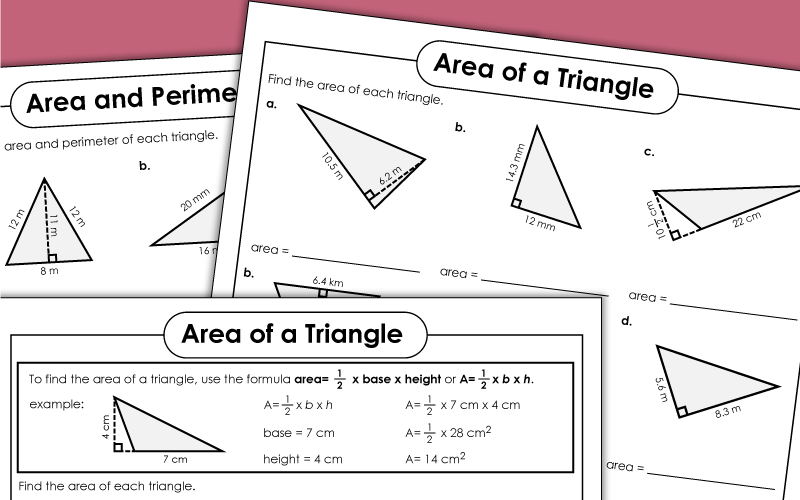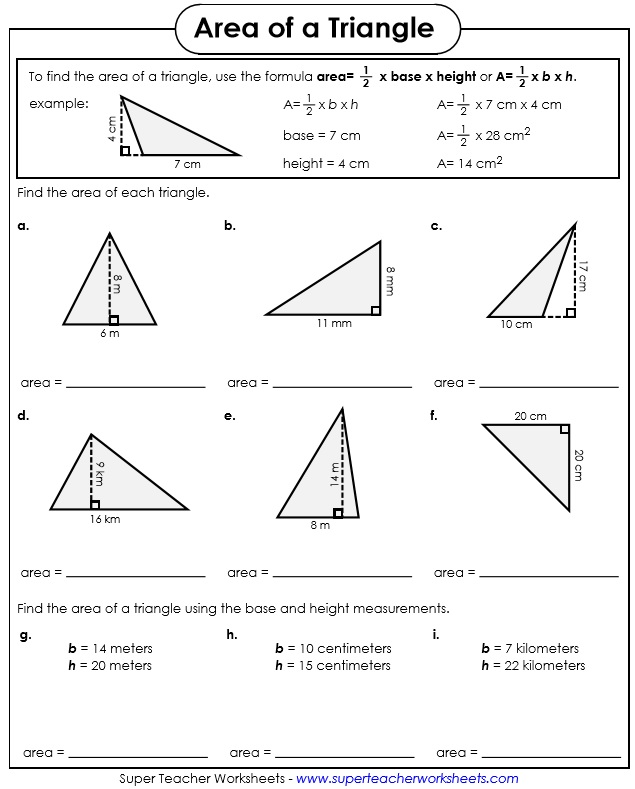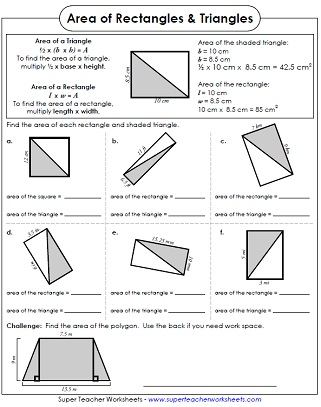# Area of Triangles

Calculate the areas of triangles on these printable worksheets.## Level: BasicSingle-Digit Measurements

Calculate the area of each of the nine triangles shown.
This printable will help students discover the relationship between the areas of rectangles and the areas of triangles.
First, find the area of three different triangles. Then find the areas of triangles within a larger shape. Then find the height when you are given the area.
For each triangle shown, students calculate the perimeter and the area.
This file contains 30 area of a triangle task cards. Use them for classroom scavenger hunts, peer tutoring, exit slips, or on your document camera.
Use the formula to find the areas of these six right triangles.

## Level: Intermediate

This worksheet shows students how to find the area of a triangle. There are 9 practice problems. Triangle measurements have two-digit numbers, decimals, and fractions.
Find the area of each of the triangles shown. This page has nine triangle illustrations and three problems with written measurements. All triangles have metric measurements.
Find the area of each rectangle. Divide each rectangle in half and find the area of each triangle. This worksheet shows the relationship between the areas of rectangles and triangles.
In the top section, your pupils will find the area of three triangles. In the middle section, find the areas of triangle parts. In the final section, they are given the area and must calculate the height.
Print these area of a triangle task cards. Use them for classroom games, exit slips, on your document camera, or scavenger hunts.

## Level: AdvancedFraction & Decimal Measurements

This worksheet shows students how to find the area of a triangle. There are 9 practice problems. Triangles measurements have mixed units, decimals, and/or fractions.
Find the areas of the triangles. Base and height measurements include larger numbers decimals, and fractions. Some triangle measurements also have mixed units.
This worksheet has three parts. In part one, students find the areas of three triangles. In part two, they find the areas of a triangle that is divided into parts. In part three, they are given the area and they calculate the height.
Find the perimeter and the area of each triangle shown. This advanced-level worksheet has 3-digit decimal numbers.
Calculate the areas of the triangles on these task cards. Use these cards for exit slips, classroom projects, or on your whiteboard document camera.

Area of Rectangles

On these worksheets, students practice finding the areas of rectangles and squares.

More Area Worksheets

Calculate the areas of rectangles and squares with the worksheets on this page. Also includes area of triangles, trapezoids, parallelograms, as well as surface area.

Geometry Worksheets

Browse our complete collection of geometry worksheets. Topics include perimeter, polygons, symmetry, tessellations, and more.

Area and Circumference of Circles

## Sample Worksheet ImagesMy Account
Site Information